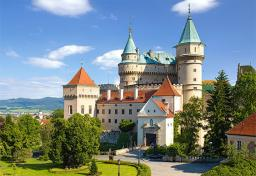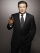# 13 tickets

A short and long sightseeing tour is possible at the castle. Ticket for a short sightseeing circuit costs CZK 60, for a long touring circuit costs CZK 100. So far, 13 tickets have been sold for 1140 CZK. How much did you pay for tickets for a short tour?

Correct result:

a =  240
b =  900

#### Solution:

a+b = 1140
a/60 + b/100 = 13

a+b = 1140
5a+3b = 3900

a = 240
b = 900

Our linear equations calculator calculates it.We would be pleased if you find an error in the word problem, spelling mistakes, or inaccuracies and send it to us. Thank you!

Showing 1 comment:Smarty
Too hard!Tips to related online calculators
Do you have a linear equation or system of equations and looking for its solution? Or do you have quadratic equation?

## Next similar math problems:

• Fifth of the numberThe fifth of the number is by 24 less than that number. What is the number?
• Linear systemSolve this linear system (two linear equations with two unknowns): x+y =36 19x+22y=720
• Football match 4In a football match with the Italy lost 3 goals with Germans. Totally fell 5 goals in the match. Determine the number of goals of Italy and Germany.
• Substitutionsolve equations by substitution: x+y= 11 y=5x-25
• Hotel roomsIn the 45 rooms, there were 169 guests, some rooms were three-bedrooms and some five-bedrooms. How many rooms were?
• BlackberriesDaniel, Jolana and Stano collected together 34 blackberries. Daniel collected 8 blackberries more than Jolana, Jolana 4 more than Stano. Determine the number blackberries each collected .
• Three figures - numbersThe sum of three numbers, if each is 10% larger than the previous one, is 662. Determine the figures.
• The largerThe larger of two numbers is nine more than four times the smaller number. The sum of the two numbers is fifty-nine. Find the two numbers.
• Vijuviju has 40 chickens and rabbits. If in all there are 90 legs. How many rabbits are there with viju??
• Family parcelsIn father will he divided the land so that the older son had three bigger part than younger son. Later elder son gave 2.5 ha field to younger and they had both the same. Determine the area of family parcel.
• Dining roomThe dining room has 11 tables (six and eight seats). In total there are 78 seats in the dining room. How many are six-and eight-seat tables?
• ATC campThe owner of the campsite offers 79 places in 22 cabins. How many of them are triple and quadruple?
• Equations - simpleSolve system of linear equations: x-2y=6 3x+2y=4
• Football seasonDalibor and Adam together scored 97 goals in the season. Adam scored 9 goals more than Dalibor. How many goals scored each?
• ButtonsMen's shirt has sewn 9 buttons and blouse 3 buttons. Together they sewed 60 pieces and consumed 390 buttons. How many shirts?
• Three brothersThe three brothers have a total of 42 years. Jan is five years younger than Peter and Peter is 2 years younger than Michael. How many years has each of them?
• SummerjobThree students participated in the summerjob. Altogether they earn 1780, -. Peter got a third less than John and Paul got 100,- more than Peter. How much got every one of them?